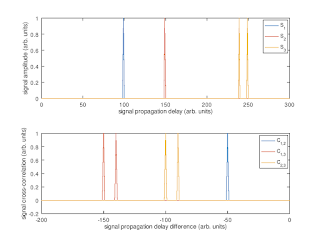## (1) Finding the common propagation mode

Let's consider the case of three receivers receiving the same signal.
• The cross-correlations produce time differences Δt1,2, Δt1,3, Δt2,3.
• Trivially, Δt1,2 = Δt1,3 - Δt2,3 (and cyclically) (*)
This situation is illustrated in the plot below.Common propagation mode

As a second step let's consider the situation when one of the three receivers receives the common signal via two propagation paths, and the cross-correlations to the signals received at the two other receivers have approximately the same magnitude.

Currently the TDoA software performs for each cross-correlation a clustering algorithm, and in the situation shown in the plot below it may well be that the following time differences are obtained: Δt1,2 = -50, Δt1,3 = -100, Δt2,3 = -140. Note that these do not fulfill the identity (*).Two propagation modes for one receiver

As a consequence, a trivial stage of pre-processing is given by selecting those cross-correlation peaks which are consistent according to (*).

## (2) Using constraints (number of degrees of freedom)

Now let us count the number of degrees of freedom for the case of N receivers receiving the same signal: there are
• 2 unknowns, (lat,lon), for the most likely position
• N unknown virtual heights of which only (N-1) are observable in the time differences (adding a common time offset does not change Δti,j)
• N(N-1)/2 hyperbolæ (=constraints)
Note that instead of virtual heights we might as well add time offsets δt to the time differences, Δti,j+δtj, j≠1, and in this way become agnostic w.r.t. the specifics of ionospheric propagation.

As a summary there are N+1 unknowns and N(N-1)/2 constraints:

3 2(pos)+2(δtj, j≠1) 3 hyperbolæ -1
4 2(pos)+3(δtj, j≠1) 6 hyperbolæ 1
N 2(pos)+(N-1)(δtj, j≠1) N(N-1)/2 hyperbolæ N(N-1)/2-N-1

So for 3 receivers there is a 1-dimensional family of solutions while for N≥4 receivers the system of equations is overdetermined.  This sounds good enough to make me suspicious if it is indeed true.

It will be interesting to try these ideas on real data obtained from KiwiSDRs. If anyone spots an error in the logic above please do let me know.

When using N stations there are N-1 independent measurements contained in the time differences δtij, j>i. Two of these are needed to determine the likely position, so N-3 out of the N-1 δti, i≠1, can be found: